# How Polynomials Behave

A polynomial looks like this:example of a polynomial

## Continuous and Smooth

There are two main things about the graphs of Polynomials:

The graphs of polynomials are continuous, which is a special term with an exact definition in calculus, but here we will use this simplified definition:we can draw it without lifting our pen from the paper

The graphs of polynomials are also smooth. No sharp "corners" or "cusps"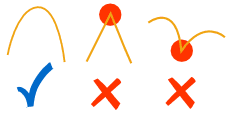## How the Curves Behave

Let us graph some polynomials to see what happens ...

f(x) = xn

Which actually does interesting things.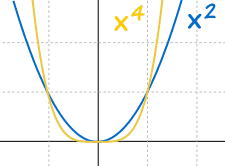Even values of "n" behave the same:

• Always above (or equal to) 0
• Always go through (0,0), (1,1) and (-1,1)
• Larger values of n flatten out near 0, and rise more sharply above the x-axis

And: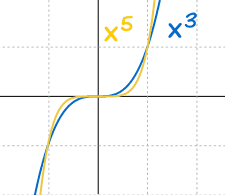Odd values of "n" behave the same

• Always go from negative x and y to positive x and y
• Always go through (0,0), (1,1) and (−1,−1)
• Larger values of n flatten out near 0, and fall/rise more sharply from the x-axis

## Power Function of Degree n

Next, by including a multiplier of a we get what is called a "Power Function":

f(x) = axn
f(x) equals a times x to the "power" (ie exponent) n

The "a" changes it this way:

• Larger values of a squash the curve (inwards to y-axis)
• Smaller values of a expand it (away from y-axis)
• And negative values of a flip it upside down
 Example: f(x) = ax2 a = 2, 1, ½, −1 Example: f(x) = ax3 a = 2, 1, ½, −1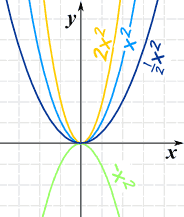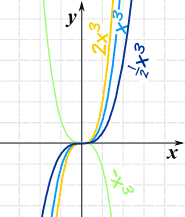We can use that knowledge when sketching some polynomials:

### Example: Make a Sketch of y=1−2x7

• We know how x3 looks,
• x7 is similar, but flatter near zero, and steeper elsewhere,
• Squash it to get 2x7,
• Flip it to get −2x7, and
• Raise it by 1 to get 1−2x7.

Like this: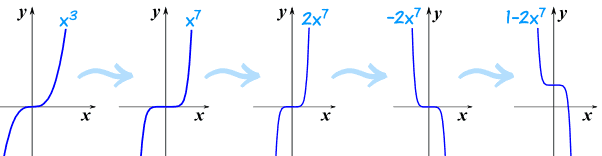So by doing this step-by-step we can get a good result.

## Turning Points

A Turning Point is an x-value where a local maximum or local minimum happens:### How many turning points does a polynomial have?

Never more than the Degree minus 1

The Degree of a Polynomial with one variable is the largest exponent of that variable.### Example: a polynomial of Degree 4 will have 3 turning points or less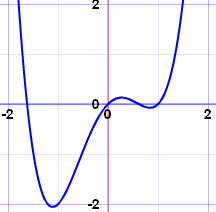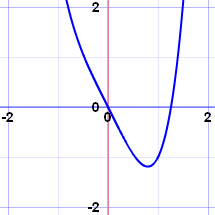x4−2x2+x has 3 turning points x4−2x has only 1 turning point

The most is 3, but there can be less.

We may not know where they are, but at least we know the most there can be!

## What Happens at the Ends

And when we move far from zero:

• far to the right (large values of x), or
• far to the left (large negative values of x)

then the graph starts to resemble the graph of y = axn where axn is the term with the highest degree.

### Example: f(x) = 3x3−4x2+x

Far to the left or right, the graph will look like 3x3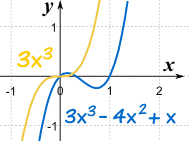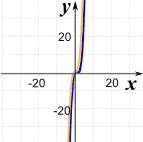Near Zero, they are different Far From Zero, they become similar

This makes sense, because when x is large, then x3 is much greater than x2 etc

This is officially called the "End Behavior Model".

And yes, we have come to the end!

## Summary

• Graphs are continuous and smooth
• Even exponents behave the same: above (or equal to) 0; go through (0,0), (1,1) and (−1,1); larger values of n flatten out near 0, and rise more sharply.
• Odd exponents behave the same: go from negative x and y to positive x and y; go through (0,0), (1,1) and (−1,−1); larger values of n flatten out near 0, and fall/rise more sharply
• Factors:
• Larger values squash the curve (inwards to y-axis)
• Smaller values expand it (away from y-axis)
• And negative values flip it upside down
• Turning points: there are "Degree − 1" or less.
• End Behavior: use the term with the largest exponent